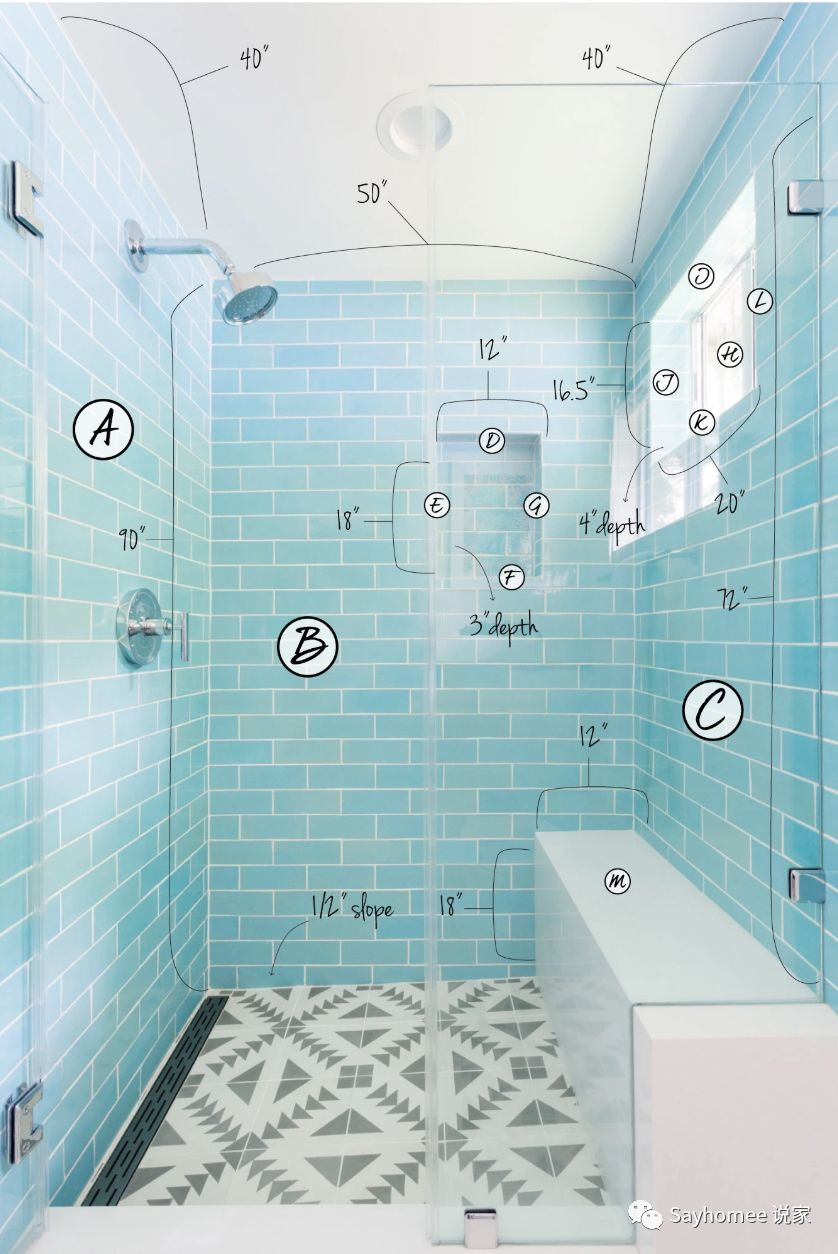# 家装中如何计算洗手间瓷砖需求#### 继续阅读? 免费订阅，及时获取更多家装信息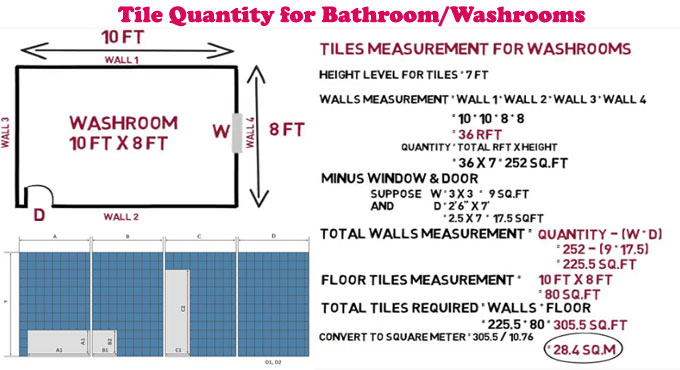• 纸张
• 书写用具
• 计算器
• 卷尺
• 空间照片（可选

#### 具体步骤

• 绘制或打印该空间的照片。
• 以英寸为单位测量每边并标记图形或照片。
• 如果需要，请将空格分成几部分，并用AZ的大写字母标记每个空格，以免混淆。
• 将每个部分的高度和宽度相乘。
• 将所有部分加在一起，然后将该数字除以144，得到平方英尺。
• 从总数中减去窗或长凳。
• 增加10％的超量。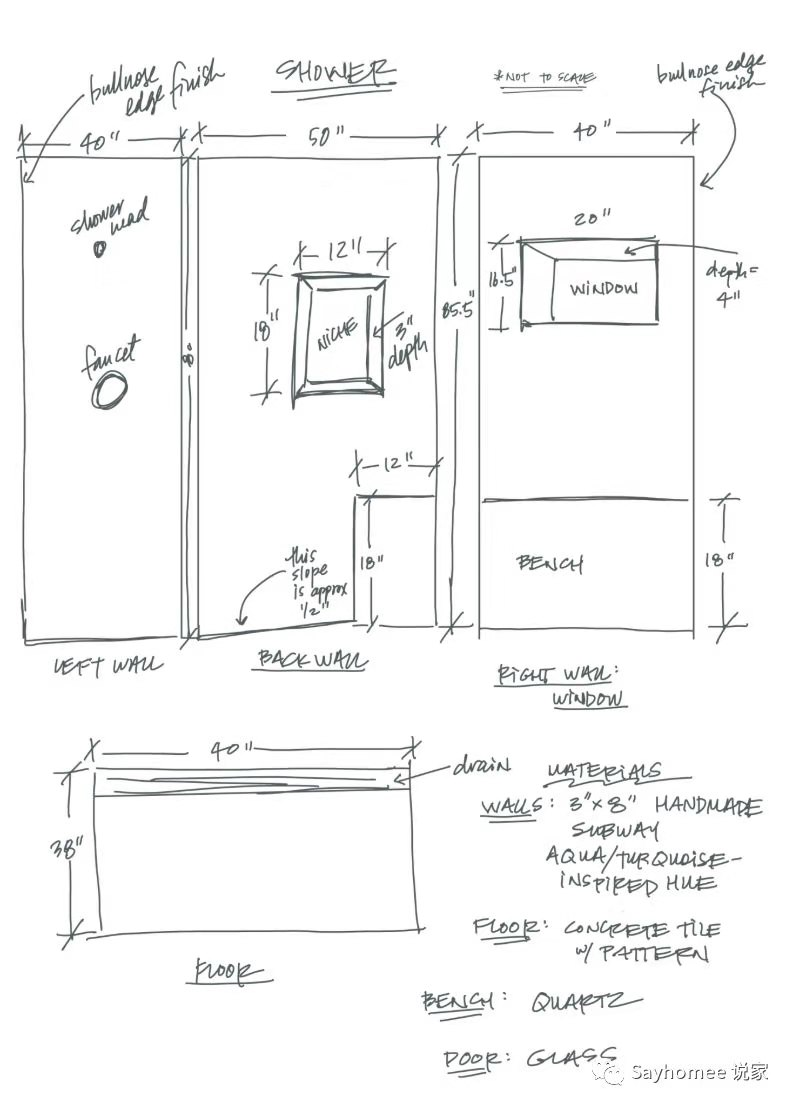##### 范例一：地板

A

• 总面积：36“ x 36” = 1,296
• 换算成平方英尺：1,296 / 144 = 9平方英尺
• （加上超量）9x1.2 = 10.8平方英尺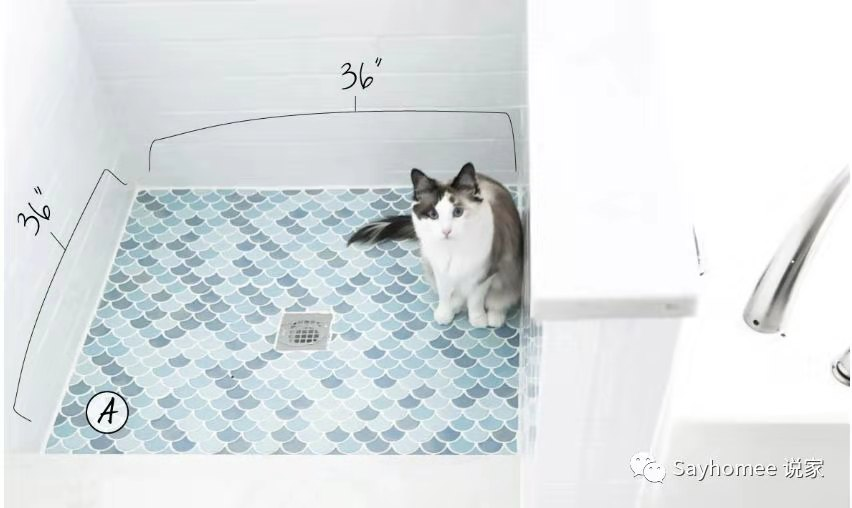##### 范例二：浴室后挡板

A

• 15.25“ x33.125” = 505.16

B

• 4.75“ x67” = 318.25

C

• 15.25“ x33.125” = 505.16

• A节+ B节+ C节= 1,328.57
• 1,328.57 / 144 = 9.2平方英尺
• （加上超量）9.2x1.1 = 10.12平方英尺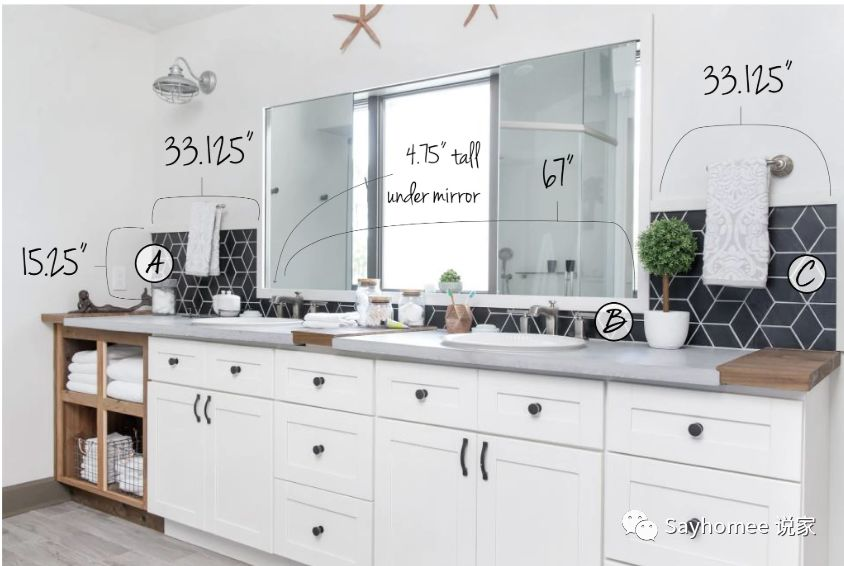##### 范例三：淋浴如何计算

 A节90“ x40” = 3,600“ H节16.5“ x20” = 330“ B区90“ x50” = 4,500“ 第一节20“ x4” = 80“ C区72“ x40” = 2,880“ J节16.5“ x4” = 66“ D区12“ x3” = 36“ K节20“ x4” = 80“ E节18“ x3” = 54“ L节16.5“ x4” = 66“ F节12“ x3” = 36“ M部分18“ x12” = 216“ G节18“ x3 = 54”

• 添加除工作台和窗户以外的所有部分 （M和H部分）= 11,452
• 现在减去没有瓷砖的区域（M和H节）：11,452-330 -216 = 10,906
• 10,906“ / 144 = 75.7平方英尺
• 75.7x1.1 = 83.3平方英尺